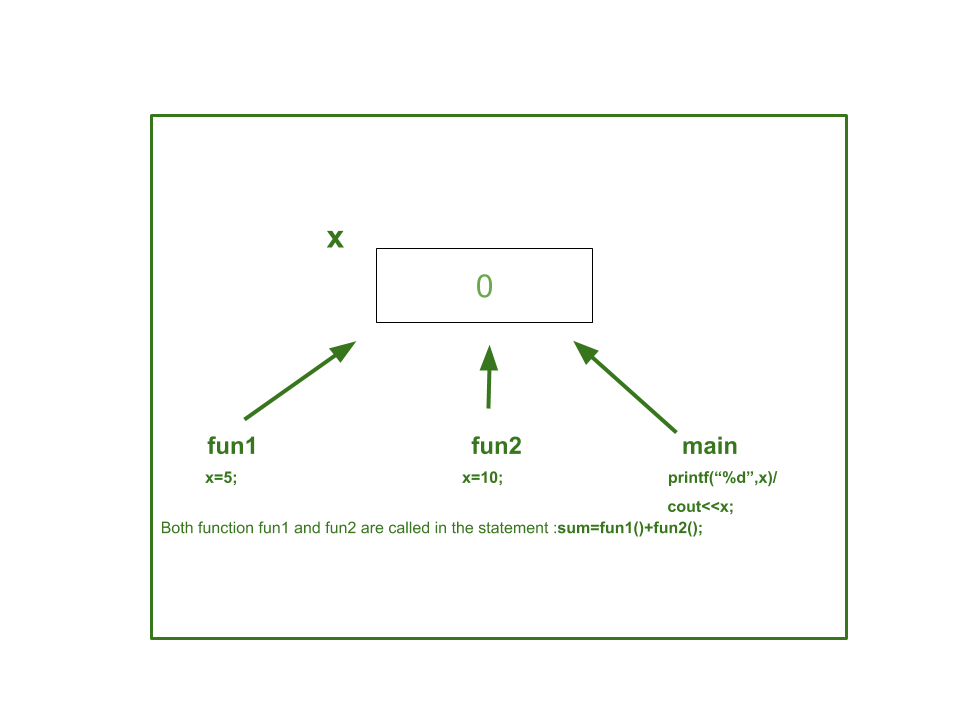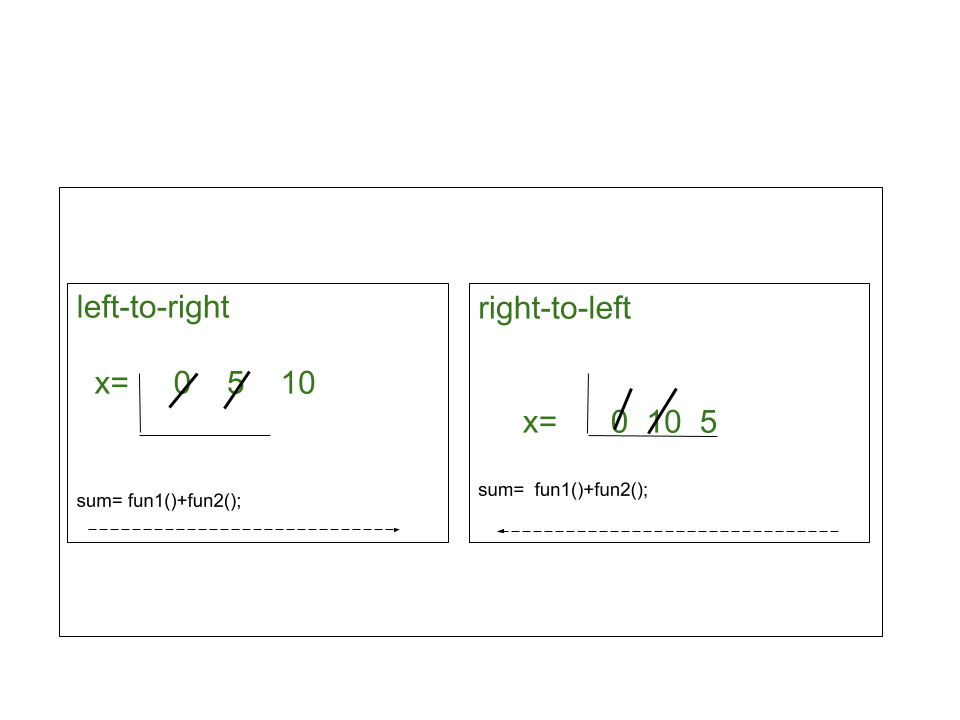Unspecified behavior in C/C++ with Examples

• Last Updated : 07 Aug, 2020

Unspecified Behavior terminology is used by users of C and C++. It is defined as Annex-J of C11 standard. Let us learn about this terminology in depth.

Type 1. Evaluation order of function arguments:

A program is said to have an unspecified behavior when the standard provides two or more possibilities but does not impose requirements on which should be chosen by the compiler writer. For Example, the order in which function fun1 and fun2 are called in the following expression is not specified!

x = fun1() + fun2();

The compiler can choose to implement it from left-to-right or right-to-left, resulting in either fun1 or fun2 being called first. The standard doesn’t detect the order of call.

Undefined Behavior results in unpredicted behavior of the entire program. But in unspecified behavior, the program makes choice at a particular junction and continue as usual like originally function executes.

Below is the program to illustrate Unspecified Behaviour:

C

 // C program to illustrate Unspecified// Behaviour#include   // Declaring x as a global variable// defining fun1int x;  // Defining function fun1()int fun1(){    x = 5;    return 2;}  // Defining function fun2()int fun2(){    x = 10;    return 3;}  // Driver Codeint main(){    // Function call    int sum = fun1() + fun2();      // Printing the value of x    printf("%d", x);    return 0;}

C++

 // C++ program to illustrate Unspecified// Behaviour#include using namespace std;  // Declaring x as a global variable// defining fun1int x;  // Defining function fun1()int fun1(){    x = 5;    return 2;}  // Defining function fun2()int fun2(){    x = 10;    return 3;}  // Driver Codeint main(){    // Function call    int sum = fun1() + fun2();      // Print the value of x    cout << x;    return 0;}
Output:
10

Explanation:

In the above program initially, x is 0. fun1() changes x to 5 and return 2. fun2() changes x to 10 and return 3. Value of sum is definitely 5, x is a global variable and all the three functions access the same global x.if a function is changing x, it is changing the same copy of x that other access.The two function calls are operands of +(add) operator if plus operator evaluates its operands from left-to-right, then fun1 will be called first setting the value of x-5, then fun2 will be called setting the final value of x as 10.

Similarly, if the order of evaluation is right to left then the final value of x will be 10 as in fun1 will be called last, and the value of x in fun1 is 5.Type 2. Value of an out-of-range enum:

If a scoped enum is converted to an integral type that is too small to hold its value, the resulting value is unspecified. Also, if an integer is converted to an enum and the integer’s value is outside the range of the enum’s values, the resulting value is unspecified.

Example:

enum Geeks {
ONE = 1,
TWO = 2,
THREE = 3,
};

Geeks s = static_cast<Geeks>(4);

However, in the next example, the behavior is not unspecified, since the source value is within the range of the enum, although it is unequal to all enumerators:

enum Geeks {
ONE = 1,
TWO = 2,
FOUR = 4,
};
Geeks s = static_cast<Geeks>(3);

Here s will have the value 3, and be unequal to ONE, TWO, and FOUR.

Below is the illustration of the same:

C++

 // C++ program to illustrate the value// of an out-of-range enum:#include using namespace std;  // enum Structureenum Geeks {    ONE = 1,    TWO = 2,    FOUR = 4,};  // Driver Codeint main(){      Geeks s = static_cast(3);      // Printing the value of s.    cout << s;    return 0;}
Output:
3

Type 3.Static cast from void* value:

If a void* value is converted to a pointer to object type, A*, but is not properly aligned for A, the resulting pointer value is unspecified.

Example:

// Suppose that alignof(int) is 4
int x = 20;
void* p1 = &x;

// Perform some pointer arithmetic...
void* p2 = static_cast<char*>(p1) + 2;
int* p3 = static_cast<int*>(p2);

The value of p3 is unspecified because p2 cannot point to an object of type int; its value is not a properly aligned address.

Type 4. Result of reinterpret_cast conversions:

The results of a reinterpret_cast from one object pointer type to another or one object reference type to another is unspecified.

Example:

int x = 42;
char* p = reinterpret_cast<char*>(&x);

However, with most compilers, this was equivalent to static_cast<char*>(static_cast<void*>(&x)) so the resulting pointer p pointed to the first byte of x. This was made the standard behavior in C++11.

C++

 // C++ program to illustrate the result// of reinterpret_cast conversions#include using namespace std;  // Driver Codeint main(){      int x = 42;    char* p = reinterpret_cast(&x);      // Print the value of p    cout << p;    return 0;}
Output:
*

Type 5. Result of some pointer comparisons:

If two pointers are compared using <, >, <=, or ≥, the result is unspecified in the following cases:

• The pointers point into different arrays i.e., A non-array object is considered an array of size (1). Below is the illustration of the same:

C++

 // C++ program to illustrate that the// pointers point into different arrays#include using namespace std;  // Driver Codeint main(){      int x;    int y;      // unspecified    const bool b1 = &x < &y;    int a;      // Given True    const bool b2 = &a < &a;      // unspecified    const bool b3 = &a < &x;      // a + 10 points past the end of array    const bool b4 = (a + 9) < (a + 10);      cout << b1 << "\n"         << b2         << "\n"         << b3 << "\n"         << b4;    return 0;}
Output:
1
1
0
1

• The pointers point into the same object, but to members with different access control. Below is the implementation of the same:

C++

 // C++ program to illustrate that the// pointers point into the same object,// but to members with different// access control#include using namespace std;  // Class Aclass A {public:    int x;    int y;      // Function returns true if    // x comes before y    bool f1() { return &x < &y; }      // unspecified    bool f2() { return &x < &z; }private:    int z;};  // Driver Codeint main(){      // Object of class A    A a;      // Function Call    cout << a.f1() << "\n"         << a.f2();    return 0;}
Output:
1
1

There are many unspecified behaviors of C language and some of them are:

1. The manner and timing of static initialization.
2. The termination status returned to the hosted environment if the return type of main is not compatible with int.
3. The value of padding bytes when sorting values in structures or unions.
4. The order in which subexpressions are evaluated and the order in which side effects take place, except as specified for the function-call ( ), &&, ||, ?:, and the comma operator.
5. the layout of storage for function parameters on the stack frame.
6. The results of rounding when the value is out of bound.
We provide nothing but the best curated videos and practice problems for our students. Check out the C Foundation Course and master the C language from basic to advanced level. Wait no more, start learning today!

My Personal Notes arrow_drop_up